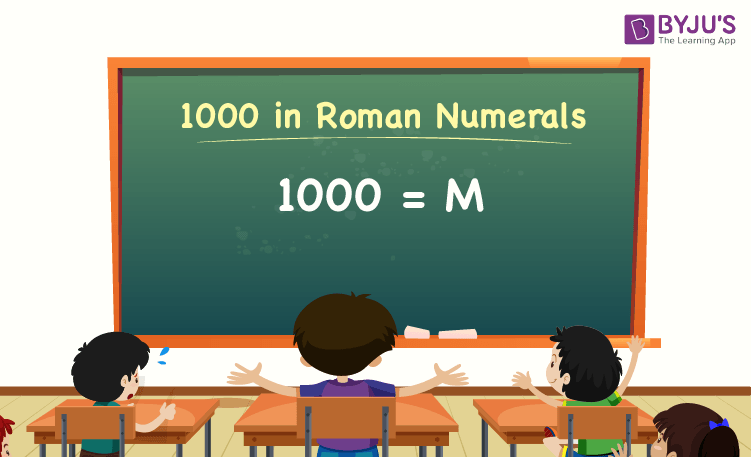# 1000 in Roman Numerals

The number 1000 in Roman numerals is M. Roman numerals, as we all know, are a way for representing numbers using Roman alphabets. Let us look at how to write the number 1000 in Roman numerals with numerous examples and solutions in this article.

 1000 in Roman Numerals = M

## How to Write 1000 in Roman Numerals?We can directly denote 1000 with the Roman numeral “M” to write it in Roman numerals. There is no expanding technique for writing 1000 in Roman numerals that allows us to perform addition or subtraction.

As a result, the Roman numeral for 1000 is M.

### Roman Numerals Related to the Number 1000

Some of the Roman numerals related to the number 1000 are as follows:

Roman Numeral for 1000 = M

Roman Numeral for 1001 = 1000 + 1 = M + I = MI

Roman Numeral for 1002 = 1000 + 2 = M + I + I = MII

Roman Numeral for 1003 = 1000 + 3 = M + I + I + 1= MIII

Roman Numeral for 1004 = 1000 + 4 = M + IV = MIV

Roman Numeral for 1005 = 1000 + 5 = M + V = MV

Roman Numeral for 1006 = 1000 + 6 = M + V + I = MVI

Roman Numeral for 1007 = 1000 + 7 = M + V + I + I = MVII

Roman Numeral for 1008 = 1000 + 8 = M + V + I + I + I = MVIII

Roman Numeral for 1009 = 1000 + 9 = M + IX = MIX

## Video Lesson on Roman Numerals### 1000 in Roman Numerals – Solved Examples

Example 1:

What is the value of 1020 – 20? Express the value in Roman numerals.

Solution:

The value of 1020 – 20 is 1000.

Thus, 1000 in Roman numerals is M.

Example 2:

Find the sum of M and C. Express the sum in Roman numerals.

Solution:

We know that,

M = 1000

C = 100

So, M + C = 1000 + 100 = 1100.

Hence, 1100 in Roman numerals is MC.

Keep visiting BYJU’S – The Learning App, and download the app to quickly understand all Maths concepts by watching more learning videos.

## Frequently Asked Questions on 1000 in Roman Numerals

Q1

### What is 1000 in Roman numerals?

1000 in Roman numerals is M.

Q2

### Express the value of 950 + 50 in Roman numerals.

The value of 950 + 50 is 1000. Hence, 1000 in Roman numerals is M.

Q3

### What should be added to 800 to get the Roman numerals M? Express the value in words.

200 should be added to 800 to get the Roman numeral M, which is 1000. Hence, 200 in Roman numerals is CC.

Test your Knowledge on 1000 in Roman Numerals